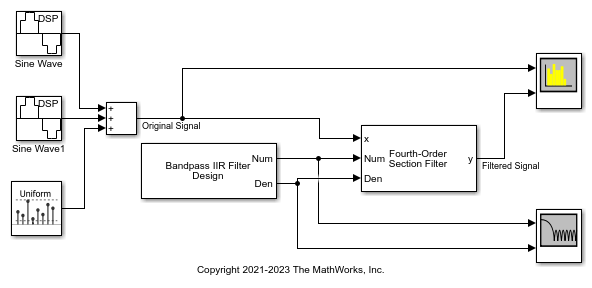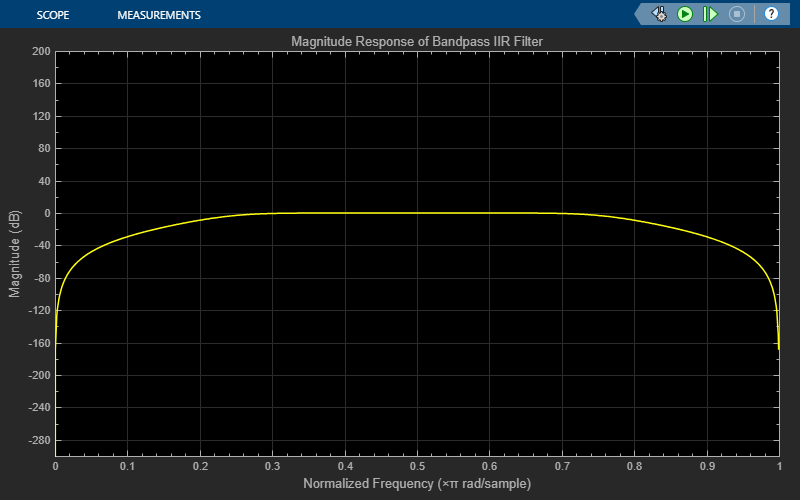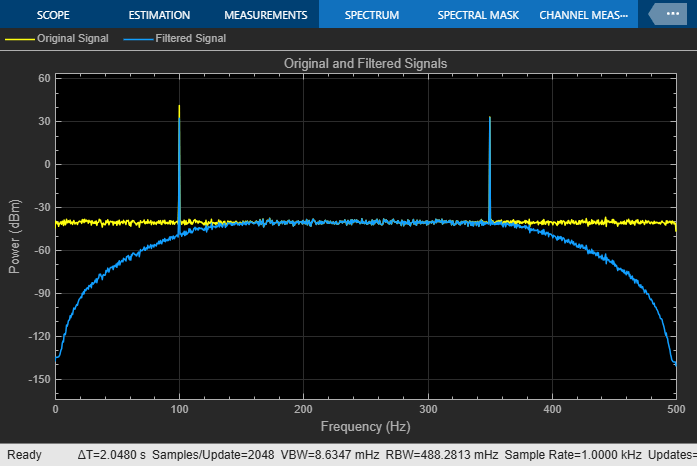# Filter Noisy Signal Using Fourth-Order Section (FOS) Filter in Simulink

Filter a noisy sinusoidal signal using the Fourth-Order Section Filter block. The numerator and denominator coefficients of the fourth-order section filter are obtained using the Tunable Bandpass IIR Filter block. You can tune the frequency specifications of this filter during simulation.

### Open and Run the Model

The input signal is a sum of two sine waves with the frequencies of 100 Hz and 350 Hz. The sample rate is 1000 Hz and the number of samples in each frame is 1024. Add zero-mean white Gaussian noise with a variance of 1e-4 to the sum of the sine waves.

The Tunable Bandpass IIR Filter block designs a sixth order bandpass IIR filter with the first and second 3-dB cutoff frequencies at 0.25rad/sample and 0.75rad/sample, respectively. Visualize the frequency response of the filter using Filter Visualizer.Filter the noisy sinusoidal signal using the Fourth-Order Section Filter block. Visualize the original sinusoidal signal and the filtered signal using the Spectrum Analyzer.### Tuning Frequency Specification of FOS Filter

During simulation, you can tune the frequency specifications of the FOS filter by tuning the frequency parameters in the Tunable Bandpass IIR Filter block. The filter response updates accordingly.

Change the first 3-dB cutoff frequency to 0.65rad/sample in the Tunable Bandpass IIR Filter block. The first tone of the sinusoidal signal is attenuated as it no longer falls in the passband frequency of the filter.